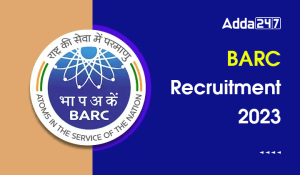Engineering Jobs   »   Mechanical Engineering Quiz

# UPRVUNL’21 ME: Daily Practices Quiz. 20-Aug-2021

Quiz: Mechanical Engineering
Exam: UPRVUNL
Topic: Thermodynamics

Each question carries 1 mark
Negative marking: 1/4 mark
Time: 8 Minutes
Q1. A closed thermodynamic system is one in which
(a) there is no energy or mass transfer across the boundary
(b) there is no mass transfer, but energy transfer exists
(c) there is no energy transfer, but mass transfer exists
(d) both energy and mass transfer take place across the boundary, but the mass transfer is controlled by valves

Q2. Which one of the following thermodynamic processes approximates the steaming of food in a pressure cooker?
(a) isenthalpic
(b) isobaric
(c) isochoric
(d) isothermal

Q3. For a simple closed system of constant composition, the difference between the net heat and work interactions is identifiable as the change in
(a) Enthalpy
(b) Entropy
(c) Flow energy
(d) Internal energy

Q4. A closed system undergoes a process 1-2 for which the values of Q_(1-2) and W_(1-2) are + 30kJ and + 60kJ, respectively. If the system is returned to state 1 from state 2 and Q_(2-1) is –20kJ, what is the value of the work W_(2-1) ?
(a) +20 kJ
(b) -50 kJ
(c) -80 kJ
(d) +40 kJ

Q5. Consider the following statements:
A real gas obeys perfect gas law at very
1. high temperatures
2. high pressures
3. low pressures
Which of these statements is/are correct?
(a) 1 only
(b) 1 and 3
(c) 2 only
(d) 3 only

Q6. An engine working on Carnot cycle rejects 40% of absorbed heat from the source, while the sink temperature is maintained at 127°c, then the source temperature is
(a) 750°C
(b) 477°C
(c) 203°C
(d) 67.5°C

S1. Ans.(b)
Sol. A closed system consists of fixed amount of mass and no mass can cross its boundary but energy in the from of heat and work can cross the boundary.

S2. Ans.(c)
Sol. Volume of pressure cooker is always constant. Hence, it is isochoric process.

S3. Ans.(d)
Sol. The given statement is for first law of Thermodynamics i.e.
δQ=dU+δW
δQ-δW=dU

S4. Ans.(b)
Sol. For process 1 -2
U_1+Q=U_2+W
U_1-U_2=W-Q=60-30=30 kJ
For process 2 – 1
U_2+Q=U_1+W
Q+U_2-U_1=W
-20-30=W
W=-50 kJ

S5. Ans.(b)
Sol. A real gas starts behaving as ideal gas at low pressure and high temperature.

S6. Ans.(b)
Sol. Sink temperature: T_2=127°C = 127 + 273 = 400K
It is given that engine rejects 40% of absorbed heat from the source
For a Carnot cycle engine

Q_1/T_1 =Q_2/T_2
Q_1/T_1 =(0.4 Q_1)/400
⇒T=400/0.4=1000 K
= 727°C

Sharing is caring!

•GATE Result 2023 Out, Download Result, C...
•GATE 2023 Admit Card Out, Download Link ...
•Daily Practice Questions PDF For Enginee...
•BARC Previous Year Question Papers, Down...
•BARC Salary 2023, Check Perks and Allowa...
•BARC Recruitment 2023, Notification, Vac...
•ISRO Scientist Recruitment 2023 Out, App...
•IB JIO Recruitment 2023 Out, Direct Link...